# Quant Finance Notebook¶

Let's delve into some finance theory!

There are several components to building a successful quantitative strategy. After the initial research phase where you want to avoid statistical fallacies like overfitting, you will need to build out 3 components:

2. a sizing strategy based on your edge
3. an execution algorithm consistent with your strategy and integrated with your brokers

Then, you will also need to a way to monitor the strategy and phase it out as the signal decays.

Today, we will take a quick look at how sizing works. There are certain theoretical sizing strategies that can be very useful as references. Let's take a look at the sensibility of using these in practice, and see how sizing usually works. We will start at the famous Kelly's Criterion and try to generalize it to be more applicable to our use case. In particular, let's try to generalize to find optimal sizing when losses can be partial (not total) and there may be multiple outcomes. This general solution may be messy to solve algebraically, but we can happily approximate it by running simulations (yay python!).

Here are some libraries we will be using:

In :
%pylab inline
matplotlib.style.use('ggplot')

import pandas as pd
import numpy as np
from scipy.stats import rv_discrete, gmean
from scipy.optimize import minimize_scalar

Populating the interactive namespace from numpy and matplotlib


### Kelly's Criterion¶

As a refresher, here is the simple Kelly's Criterion from Wikipedia, which tells you what is the optimal amount to bet to maximize your return:

$$f = \frac{bp - q}{b} = \frac{p(b + 1) - 1}{b}$$

where:

• f is the fraction of the current bankroll to wager, i.e. how much to bet;
• b is the net odds received on the wager ("b to 1"); that is, you could win \$b (on top of getting back your \$1 wagered) for a \$1 bet • p is the probability of winning; • q is the probability of losing, which is 1 − p. Let's translate bets into the more familiar context and terms for a stock: $$\text{Amount Risked} = \frac{bp - q}{b} = \frac{bp + (-1)q}{b} = \frac{ \text{Expected Return} }{\text{Upside}}$$ And in the scenario of say a stock, we don't always lose everything that we wager. Let's instead setup the following example of a trade with a binary result: In : upside = +0.01 # return if we make money on the trade downside = -0.03 # return if we lose money on the trade prob = 0.77 # probability of making money for this trade  This could for example be the result of buying S&P 500 for a day. Let's calculate Kelly's Criterion where you only lose the downside instead of the whole wager: In : expected_return = upside * prob + downside * (1 - prob) risk_allocation = expected_return / upside notional_allocation = risk_allocation / -downside print(f"Kelly's Criterion says you should risk at a maximum {risk_allocation:.1%} of your portfolio, " f"which means buying stock with a notional amount worth around {notional_allocation :.2}x of your portfolio value.\n" f"Using this sizing, the trade will have a$-EV of +${notional_allocation * 100 * expected_return:.2} for a$100 portfolio.")

Kelly's Criterion says you should risk at a maximum 8.0% of your portfolio, which means buying stock with a notional amount worth around 2.7x of your portfolio value.
Using this sizing, the trade will have a $-EV of +$0.21 for a $100 portfolio.  Great- this checks out with our real world knowledge. For a day trader (who doesn't hold any positions overnight and take on overnight risk), you do often take on intraday positions greater than your portfolio value. And if we were really expecting to be make >20bps per day on a trading strategy, this is probably a pretty good trade that deserves biggish sizing. Okay, so we have a nice theoretical value to work off of. Let's try to see if we can arrive at a similar conclusion via simulations. In general, it is useful to run simulations to check if we get similar results, so that we can be more confident that we have not made any errors in our theoretical calculations. ### Monte Carlo Simulations¶ First, let's try to put this return distribution into a scipy random variable. Interestingly, the constructor for a discrete random variable in scipy expects a pmf with integer outcomes. If you pass in floating point values, it doesn't error but gives really weird results at some point when the values get round down / assumed to be ints. Let's pass in the upside/downside as a percentage for now to deal with this. In : binary_trade = rv_discrete(values=( [int(upside * 100), int(downside * 100)], [prob, 1 - prob] ))  To double check that everything is working, we can plot the cdf: In : graph_range = np.arange(-1, 1, 0.01) plt.xlabel('stock return') plt.title('CDF of stock returns') plt.plot(graph_range, binary_trade.cdf(graph_range * 100))  Out: [<matplotlib.lines.Line2D at 0x7f908ed4de80>]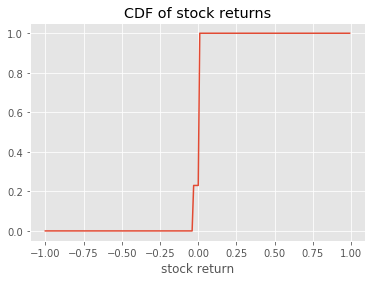And we can sample from it: In : binary_trade.rvs(size=10) / 100  Out: array([ 0.01, 0.01, 0.01, 0.01, 0.01, 0.01, 0.01, -0.03, -0.03, 0.01]) So far so good. Being able to sample from the distribution is great because this means that we can run some quick simulations where we trade with different sizes to see how each strategy turns out. In : number_of_trades = 10000 simulated_asset_returns = binary_trade.rvs(size=number_of_trades) / 100 fig, ax1 = plt.subplots() graph_data_reduction = 50 # so the graph doesn't get too big to render. only show every 50 trades on chart graph_xs = range(0, number_of_trades, graph_data_reduction) # simulate 10000 trades done using a particular sizing strategy # green lines that start dark green and turn light green start_size = 0.5 end_size = 3.0 for sizing in np.arange(start_size, end_size, 0.25): portfolio_value = (simulated_asset_returns * sizing + 1).cumprod() ax1.plot(graph_xs, portfolio_value[::graph_data_reduction], label=sizing, linestyle='--', color=(0, (sizing - start_size) / (end_size - start_size) / 1.5 + 0.33, 0)) # red lines that start light red and turn dark red start_size = 3.0 end_size = 6.0 for sizing in np.arange(start_size, end_size, 0.5): portfolio_value = (simulated_asset_returns * sizing + 1).cumprod() ax1.plot(graph_xs, portfolio_value[::graph_data_reduction], label=sizing, linestyle=':', color=((end_size - sizing) / (end_size - start_size) / 1.5 + 0.33, 0, 0)) ax1.legend(bbox_to_anchor=(1.05, 1), loc=2, borderaxespad=0.) plt.yscale('log') plt.ylabel('portfolio NAV') plt.xlabel('trades')  Out: Text(0.5,0,'trades')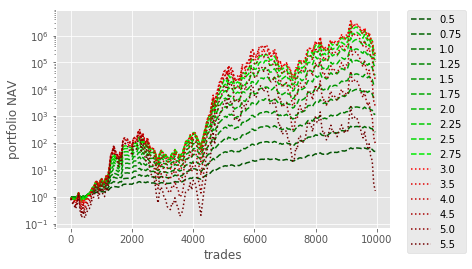Great. So we can see that our simulation supports our theoretical derivation. In particular, we see that the darker coloured lines give much lower long term returns. ie. after many trades, the total portfolio value is lower than the ligher lines. For the green lines, the dark green lines have suboptimal returns because we are not sizing large enough. For the red lines, the dark red lines have suboptimal returns because we are sizing too big (and therefore if the trade ever goes wrong and loses money, we take too big of a hit and we take forever to recover). Try running the above simulation a couple more times to see how the returns and the "optimal sizing" fluctuate! More on this later. Here is another way to reason about the optimal sizing. In : def generate_optimal_sizing_chart(simulated_returns): sizing_vs_return = [] for sizing in np.arange(0, 6.0, 0.25): sizing_vs_return.append((sizing, (simulated_asset_returns * sizing + 1).prod())) plt.scatter(*zip(*sizing_vs_return)) plt.xlabel('sizing') plt.ylabel('portfolio value') plt.yscale('log') simulated_asset_returns = binary_trade.rvs(size=number_of_trades) / 100 generate_optimal_sizing_chart(simulated_asset_returns)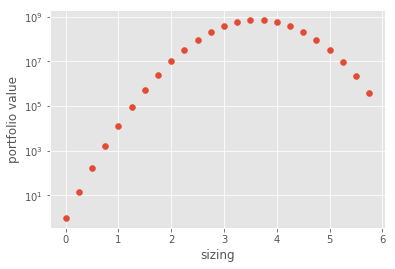Given a series of returns, we can in fact use a scipy optimization function to pinpoint which exact sizing is optimal. In : # we will try to minimize this function def money_lost(sizing): return -(simulated_asset_returns * sizing + 1).prod() simulated_asset_returns = binary_trade.rvs(size=number_of_trades) / 100 minimize_scalar(money_lost, method='bounded', bounds=(-1, 5)).x  Out: 2.226666667044402 We see that depending on the simulated asset returns, this optimal sizing fluctuates quite a bit. Let's run the simulation 1000 times and see if we can understand more about optimal sizing. In : optimization_results = [] for ii in range(100): simulated_asset_returns = binary_trade.rvs(size=number_of_trades) / 100 optimization_results.append(minimize_scalar(money_lost, method='bounded', bounds=(-1, 5)).x) results = pd.Series(optimization_results) results.describe()  Out: count 100.000000 mean 2.592800 std 0.535391 min 1.386667 25% 2.216667 50% 2.560000 75% 2.973333 max 3.893333 dtype: float64 In : results.plot.hist(bins=20)  Out: <matplotlib.axes._subplots.AxesSubplot at 0x7f908aedba20>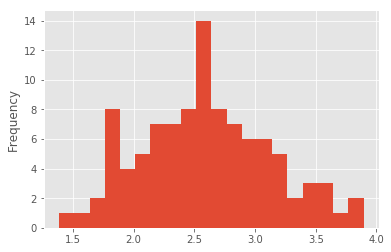Okay- so we see that the "optimal sizing" fluctuates quite a bit, but it is probably between 2-3. Further Investigation If you have time, you may want to investigate what happens if there is also a risk free alternative to trading- what if you can take whatever you did not invest, put it in the bank and make a guaranteed x% return on it? Also, if you trade more than 100% of your portfolio, you would have to pay a x% interest on that amount. How would that affect optimal sizing? I would posit that the optimal sizing is $$\text{Optimal Amount Risked} = \frac{ \text{Expected Return} - \text{Risk Free Rate} }{\text{Upside}}$$ Try to run some simulations to convince yourself! ### Practical Considerations¶ Did you noticed how easily "optimal sizing" fluctuates depending on how the returns happen to pan out for that simulation run? This is one key problem with the theoretical approach. In a world where you do not know what the exact probability distribution/mean/stdev is, the wise approach is to ere on the conservative side in practice. One arbitrary rule of thumb that some traders traditionally used is to size at half Kelly (0.5x the optimal size). We tend to veer on the safe side because of 1. Risk of ruin- sizing too big may result in us catastrophically "blowing up" 2. Path dependency- we are not risk neutral and suffer from cognitive biases. For the same final result, we much prefer getting there with lower volatility than higher volatility Let's take a look at how easily mean/stdev estimates can change and hence how much optimal sizing can fluctuate in a real world example. In : import quandl quandl.ApiConfig.api_key = 'nCnqK9fotdzGHTfUUsz1' # you should sign up for your own free API key aapl = quandl.get('WIKI/AAPL') aapl.head()  Out: Open High Low Close Volume Ex-Dividend Split Ratio Adj. Open Adj. High Adj. Low Adj. Close Adj. Volume Date 1980-12-12 28.75 28.87 28.75 28.75 2093900.0 0.0 1.0 0.422706 0.424470 0.422706 0.422706 117258400.0 1980-12-15 27.38 27.38 27.25 27.25 785200.0 0.0 1.0 0.402563 0.402563 0.400652 0.400652 43971200.0 1980-12-16 25.37 25.37 25.25 25.25 472000.0 0.0 1.0 0.373010 0.373010 0.371246 0.371246 26432000.0 1980-12-17 25.87 26.00 25.87 25.87 385900.0 0.0 1.0 0.380362 0.382273 0.380362 0.380362 21610400.0 1980-12-18 26.63 26.75 26.63 26.63 327900.0 0.0 1.0 0.391536 0.393300 0.391536 0.391536 18362400.0 A Side Note on Data Sources The library that we are using to get stock data is Quandl. They are a data aggregator that gives you a unified api to directly get data. And they return that data in pandas dataframes. Super simple, super nice. For more examples of how to use Quandl and pandas, check out this. From Quandl, you can access all kinds of data from the St Louis Fred, the commitment of traders report etc. Some of their datasets are provided free vs some are paid (eg: vendors sell data on their platform). Having a unified api means that you don't need to waste time getting your code to interface with 10 different data vendors, or to write different scrapers just to get your data. The flip side is they now control your data flow and if you need to access their api over 50k times a day, you may end up having to pay for the api access. In addition to Quandl, here are some free data sources you can consider accessing. If you do collect a lot of niche/proprietary data, the best tool for you may be scrapy. They provide a very well structured way for you to write your scrapers, meaning that you won't just be stuck with a potpourri of different scrapers- instead you will once again plug into a unified pipeline as soon as possible. There are even specialized Scraping-as-a-Service companies such as scrapy cloud that you could consider using. Otherwise, you can also just run it as a cron job/scheduled task. Back on Track Okay- let's say we use the trailing 2520 trading days (ten years) to estimate the return distribution. Let's plot a graph showing how this "optimal sizing" changes. Before we do that, we must generalize our Kelly's Criterion formula further. If you read the Wikipedia article referenced above, you would have also seen a section deriving the bet sizing for stocks assuming that stock prices follow a geometric Brownian motion: $$f = \frac{\mu - r}{ \sigma ^ 2}$$ where: •$ \mu $is the stochastic drift •$ \sigma $is the standard deviation of log-returns • r is the risk free rate In : logreturns = np.log(aapl['Close'].pct_change().dropna() + 1) logreturns.head()  Out: Date 1980-12-15 -0.053584 1980-12-16 -0.076227 1980-12-17 0.024258 1980-12-18 0.028954 1980-12-19 0.059055 Name: Close, dtype: float64 In : print(logreturns.describe()) logreturns.plot.hist(bins=20)  count 9399.000000 mean 0.000188 std 0.037377 min -1.930035 25% -0.013857 50% 0.000000 75% 0.014882 max 0.286796 Name: Close, dtype: float64  Out: <matplotlib.axes._subplots.AxesSubplot at 0x7f90897c82b0>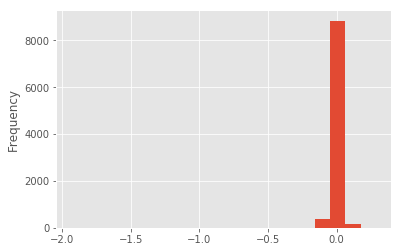Hmm- definitely looks like we have some weird outlier near -2. Let's find it. In : logreturns.loc[logreturns < -0.5]  Out: Date 1987-06-16 -0.637405 2000-06-21 -0.598870 2000-09-29 -0.731247 2005-02-28 -0.684977 2014-06-09 -1.930035 Name: Close, dtype: float64 Okay- let's dig a bit deeper to decide if we want to clean this. In : aapl['2014-01-01':'2015-01-01'].Close.plot()  Out: <matplotlib.axes._subplots.AxesSubplot at 0x7f908aedbc50>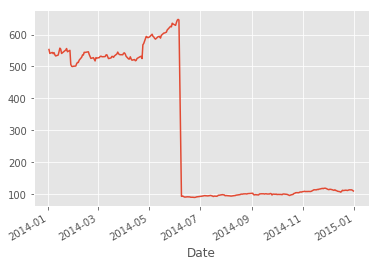Aha! There was a 7-for-1 stock split on Jun 9, 2014. There was also a 2-for-1 split on Feb 28, 2005, Jun 21, 2000, and Jun 16, 1987. Checking the news, AAPL did actually drop 52% on Sept 29, 2000 though! Let's just take out anything > 10 standard deviations from the mean. In : mean_returns = logreturns.mean() std_returns = logreturns.std() cleaned_returns = logreturns[np.abs(logreturns - mean_returns) <= (10 * std_returns)] cleaned_returns.plot.hist(bins=20)  Out: <matplotlib.axes._subplots.AxesSubplot at 0x7f908961f4e0>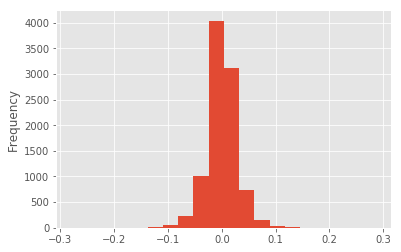In : len(logreturns) - len(cleaned_returns)  Out: 5 Okay. We did not unintentionally remove any other data. In : rolling_mean = cleaned_returns.rolling(2520, min_periods=2520).mean().dropna() rolling_std = cleaned_returns.rolling(2520, min_periods=2520).std().dropna() rolling_sizing = rolling_mean / np.square(rolling_std) fig, (ax1, ax2, ax3) = plt.subplots(3, 1, sharex=True) rolling_sizing.plot(ax=ax1, legend=True, label='Optimal Sizing', color='green') rolling_mean.plot(ax=ax2, legend=True, label='Geometric Mean') rolling_std.plot(ax=ax3, legend=True, label='Daily Vol', color='blue') original_width, original_height = fig.get_size_inches() fig.set_size_inches(original_width, original_height * 3)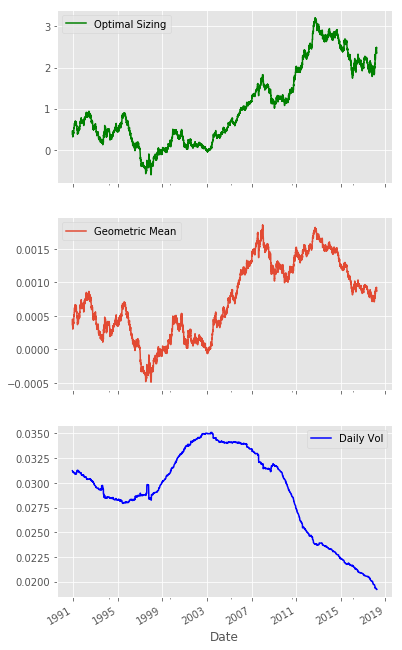A couple thoughts. We see that the "optimal sizing" really fluctuates around quite a bit. This is expected, as we are looking at a plain vanilla long stock trade without any particular edge. The returns will be a lot more noisy vs a typical quant strategy, because in the quant strategy, 1. you are purposely choosing factors that have demonstrated a stable and consistent positive return (vs here we just chose to buy a stock randomly) 2. you are likely hedging and isolating the factor from noise (eg: by hedging market beta) Let's take a look at two periods: pre-2007 and post-2007. First off, we see that optimal sizing within a one year period could change by say up to 25% of portfolio value. In the pre-2007 period, this is pretty similar to the half Kelly heuristic that some traders use. (eg: optimal sizing could have been estimated at 50%, but by the end of the year "optimal" is actually 25% if we had somehow known beforehand what was going to happen) Post-2007, we see a steady increase in optimal sizing. This is mainly driven by the vol crunch. Again, this corresponds to our knowledge of the financial markets- since 2007, central bank quantitative easing has incentivized people to lever up and take more risk. ### Further Investigations¶ • Let's say your holding period for the stock is 1 year instead of 1 day. How would that change your sizing? • We already found optimal sizing for a binary outcome and for a continuous-time stochastic process. What about a trade with three possible discrete outcomes? Let's approximate the optimal sizing by simulation! • Let's try sampling from the binary outcomes and take what we see to approximate a$ \mu $and$ \sigma $. Does the optimal sizing calculated by$ f = \frac{\mu - r}{ \sigma ^ 2} $differ from the correct optimal sizing? Now take the random variable wwith three possible discrete outcomes. How does the optimal sizing calculated by$ f = \frac{\mu - r}{ \sigma ^ 2} \$ differ with what we have found by running simulations?
• In the generate_optimal_sizing_chart function, we see long term EV vs Sizing. Add an extra feature to generate Sharpe ratio vs Sizing and overlay it on the chart. Also overlay the expected P&L of a single trade on the same chart and compare what the differences are!

Some more resources:

• fecon235 seems like a pretty cool library with lots of different ideas you would look at. Try your hand at understanding what they do, implement some strategies from there, and then
• If you enjoy learning through videos, here's a video series by Sentdex.

Some more thoughts about sizing. If you are interested in learning more about sizing, here are a few resources:

• While Turtle Traders are not quantitative traders, their thought process about "units of risk" is very enlightening and will help you develop an intuitive understanding for how this works
• Euan Sinclair's Volatility Trading also goes into great detail about how to think about volatility and possibly the definitive book I've read on measuring volatility

If you have an investment strategy with esoteric trades which do not have a typical log-normal return profile (eg: if you were a market maker, or if you were a merger arb fund), you could take a look at your past trades to (a) evaluate how accurate you were at estimating ev/risk- and hence understand how far smaller than optimal you can consider sizing, and (b) if you are putting on a similar trade, you can use the ev/risk profile of those past trades to directly estimate sizing, so you don't need to estimate it again!

We have not looked at sizing considerations on a portfolio level (ie. how trades interact with each other) in this notebook. For that, check out MPT and Black-Litterman. If you are in a pinch (or if you want to simplify things, or if you want to stress test your portfolio), you can also consider sizing your portfolio risk level with Kelly Criterion calculations discussed here and assuming all risk assets have correlation gone to 1.